## Agenda

• Simulating from distributions
• Quantile transform method
• Rejection sampling

## Simulation

Why simulate?

• We want to see what a probability model actually does
• We want to understand how our procedure works on a test case
• We want to use a partly-random procedure

All of these require drawing random variables from distributions

## Simulation

We have seen R has built in distributions: beta, binom, cauchy, chisq, exp, f, gamma, geom, hyper, logis, lnorm, nbinom, norm, pois, t, tukey, unif, weibull, wilcox, signrank

Every distribution that R handles has four functions.

• p for "probability", the cumulative distribution function (c. d. f.)
• q for "quantile", the inverse c. d. f.
• d for "density", the density function (p. f. or p. d. f.)
• r for "random", a random variable having the specified distribution

## Simulation

• Usually, R gets Uniform$$(0,1)$$ random variates via a pseudorandom generator, e.g. the linear congruential generator
• Uses a sequence of Uniform$$(0,1)$$ random variates to generate other distributions
• How?

## Example: Binomial

• Suppose we want to generate a Binomial$$(1,1/3)$$ using a $$U \sim \text{Uniform}(0,1)$$
• Consider the function $$X^* = I(0<u<1/3)$$, then $P(X^* = 1) = P( I(0<u<1/3 = 1) = P( u \in (0,1/3)) = 1/3$ and $$P(X^* = 0) = 2/3$$
• Hence, $$X^* \sim \text{Binomial}(1,1/3)$$
• Two ways to extend this to Binomial$$(n,1/3)$$

## Example: Binomial

my.binom.1 <- function(n=1, p=1/3){
u <- runif(n)
binom <- sum(u<p)
return(binom)
}

my.binom.1(1000)
##  354
my.binom.1(1000, .5)
##  525

## Example: Binomial

my.binom.2 <- function(n=1, p=1/3){
u <- runif(1)
binom <- qbinom(u, size=n, prob=p)
return(binom)
}

my.binom.2(1000)
##  349
my.binom.2(1000, .5)
##  493

## Quantile transform method

Given $$U \sim \text{Uniform}(0,1)$$ and CDF $$F$$ from a continuous distribution. Then $$X = F^{-1}(U)$$ is a random variable with CDF $$F$$.

Proof: $P(X\le a) = P (F^{-1}(U) \le a) = P ( U \le F(a)) = F(a)$

• $$F^{-1}$$ is the quantile function
• If we can generate uniforms and calculate quantiles, we can generate non-uniforms
• Also known as the Probability Integral Transform Method

## Example: Exponential

Suppose $$X \sim \text{Exp}(\beta)$$. Then we have density $f(x) = \beta^{-1} e^{-x/\beta} I(0<x<\infty)$ and CDF $F(x) = 1 - e^{-x/\beta}$ Also $y = 1 - e^{-x/\beta} \text{ iff } -x/\beta = \log (1-y) \text{ iff } x = -\beta \log (1-y).$ Thus, $$F^{-1} (y) = -\beta \log(1-y)$$.

So if $$U \sim \text{Uniform}(0,1)$$, then $$F^{-1} (u) = -\beta \log(1-u) \sim \text{Exp}(\beta)$$.

## Example: Exponential

x <- runif(10000)
y <- - 3 * log(1-x)
hist(y)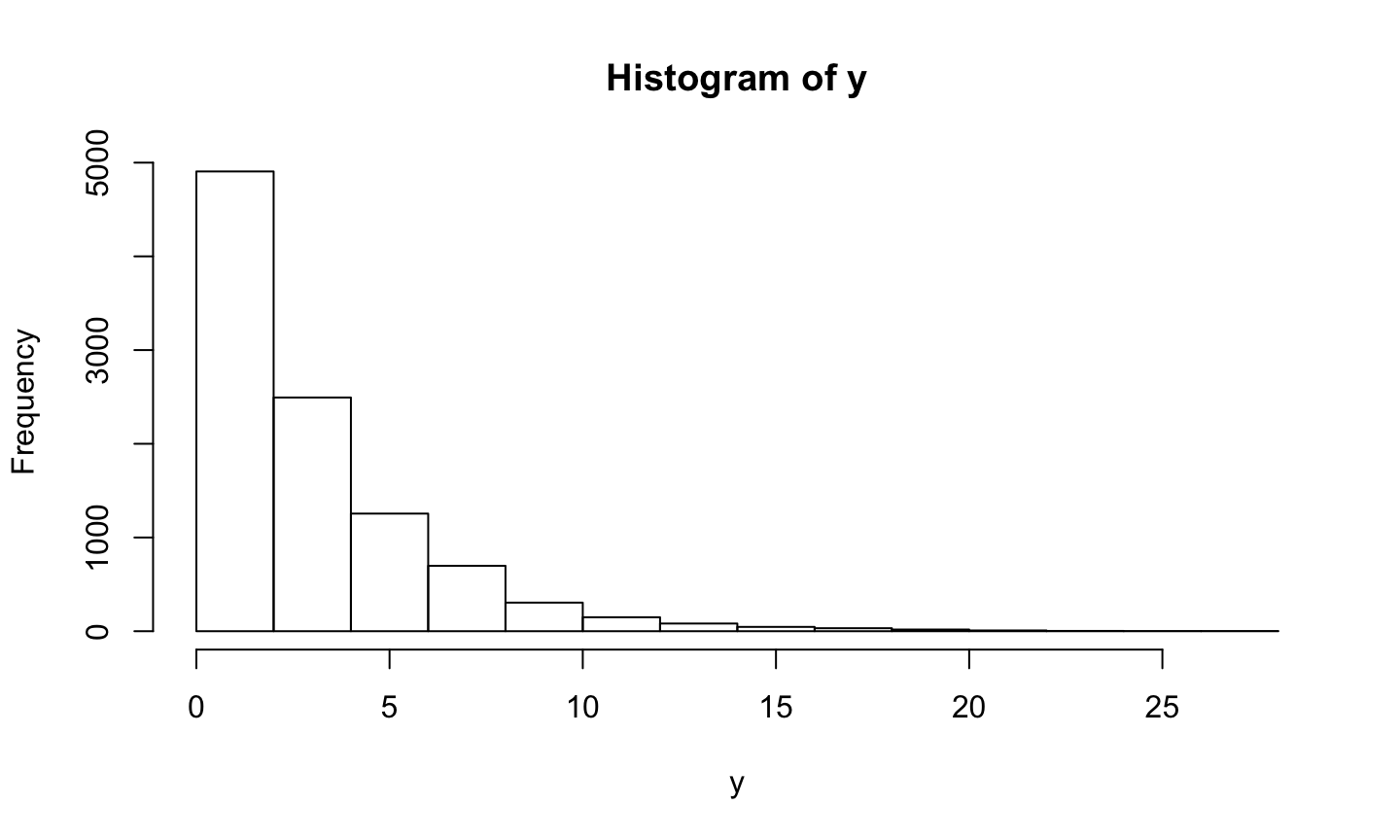mean(y)
##  2.98428

## Example: Exponential

true.x <- seq(0,30, .5)
true.y <- dexp(true.x, 1/3)
hist(y, freq=F, breaks=30)
points(true.x, true.y, type="l", col="red", lw=2)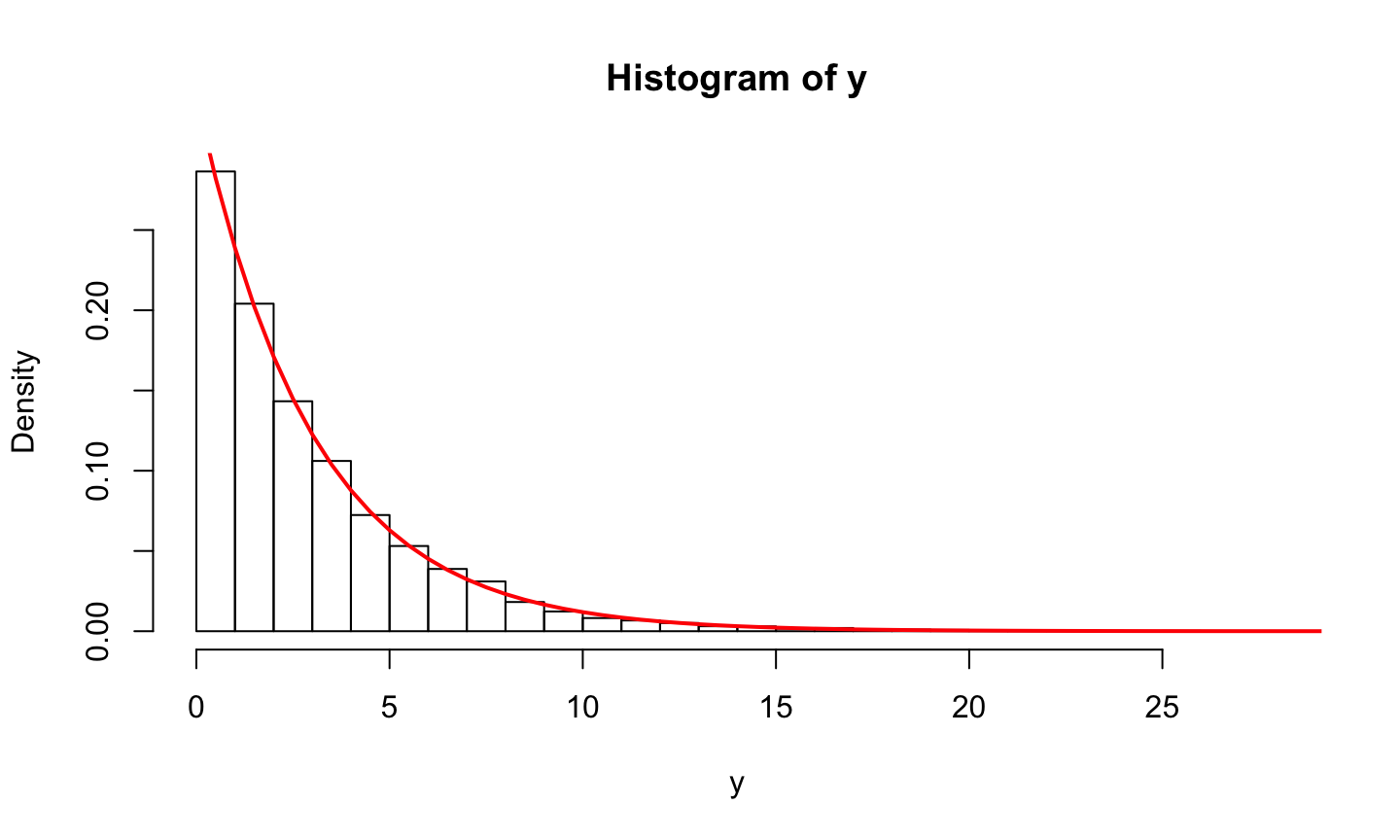## Example: Gamma

• Remember that if $$X_1, \dots, X_n$$ are IID $$\text{Exp}(\beta)$$, then $$\sum_{i=1}^n X_i \sim \Gamma(n, \beta)$$
• Hence if we need a $$\Gamma(\alpha, \beta)$$ random variate and $$\alpha \in \{ 1, 2, \dots \}$$, then take $$U_1, \dots, U_{\alpha}$$ IID $$\text{Uniform}(0,1)$$ and set $\sum_{i=1}^{\alpha} -\beta \log(1-u_i) \sim \Gamma(\alpha, \beta)$
• What if $$\alpha$$ is not an integer?

## Quantile transform method

• Quantile functions often don’t have closed form solutions or even nice numerical solutions
• But we know the probability density function — can we use that?

## Rejection sampling

• The accept-reject algorithm is an indirect method of simulation
• Uses draws from a density $$f_y(y)$$ to get draws from $$f_x(x)$$
• Sampling from the wrong distribution and correcting it

## Rejection sampling

Theorem: Let $$X \sim f_x$$ and $$Y \sim f_y$$ where the two densities have common support. Define $M = \sup_{x} \frac{f_x(x)}{f_y(x)}.$ If $$M< \infty$$ then we can generate $$X \sim f_x$$ as follows,

1. Generate $$Y \sim f_y$$ and independently draw $$U \sim \text{Uniform}(0,1)$$
2. If $u < \frac{f_x(y)}{M f_y(y)}$ set $$X=Y$$; otherwise return to 1.

Exercise: Why is $$M\ge 1$$?

## Rejection sampling

Proof: \begin{aligned} P(X \le x) & = P \left( Y \le x \; \middle| \; \text{STOP} \right)\\ & = P \left( Y \le x \; \middle| \; u \le \frac{f_x(y)}{M f_y(y)} \right) \\ & = \frac{P \left( Y \le x , u \le \frac{f_x(y)}{M f_y(y)} \right)}{P \left( u \le \frac{f_x(y)}{M f_y(y)} \right)} \\ & = \frac{A}{B} \end{aligned}

## Rejection sampling

Now, we have \begin{aligned} A & = P \left( Y \le x , u \le \frac{f_x(y)}{M f_y(y)} \right) \\ & = E \left[ P \left( Y \le x , u \le \frac{f_x(y)}{M f_y(y)} \right) \; \middle| \; y \right] \\ & = E \left[ I (y \le x) \frac{f_x(y)}{M f_y(y)} \right] \\ & = \int_{-\infty}^{\infty} I (y \le x) \frac{f_x(y)}{M f_y(y)} f_y(y) dy \\ & = \frac{1}{M} \int_{-\infty}^{x} f_x(y) dy = \frac{F_x(x)}{M} \end{aligned}

## Rejection sampling

Similarly, we have \begin{aligned} B & = P \left( u \le \frac{f_x(y)}{M f_y(y)} \right) \\ & = E \left[ P \left( u \le \frac{f_x(y)}{M f_y(y)} \right) \; \middle| \; y \right] \\ & = E \left[ \frac{f_x(y)}{M f_y(y)} \right] \\ & = \int_{-\infty}^{\infty} \frac{f_x(y)}{M f_y(y)} f_y(y) dy \\ & = \frac{1}{M} \int_{-\infty}^{\infty} f_x(y) dy = \frac{1}{M} \end{aligned}

## Rejection sampling

Hence, \begin{aligned} P(X \le x) & = \frac{A}{B} \\ & = \frac{\frac{F_x(x)}{M}}{\frac{1}{M}} = F_x(x) \end{aligned} And the proof is complete. That is, $$X \sim f_x$$.

## Rejection sampling

• Notice, $P(\text{STOP}) = B = P \left( u \le \frac{f_x(y)}{M f_y(y)} \right) = \frac{1}{M}$
• Thus the number of iterations until the algorithm stops is Geometric($$1/M$$)
• Hence, the expected number of iterations until acceptance is $$M$$.

## Example: Gamma

• Suppose we want to simulate $$X \sim \Gamma(3/2, 1)$$ with density $f_x(x) = \frac{2}{\pi} \sqrt{x} e^{-x} I(0<x<\infty).$
• Can use the accept-reject algorithm with a $$\Gamma(n,1)$$ and $$n \in \{ 1, 2, \dots \}$$ since we know how to simulate this

## Example: Gamma

• Then we have \begin{aligned} M & = \sup_{x>0} \frac{f_x(x)}{f_y(x)}\\ & = \sup_{x>0} \frac{\frac{2}{\pi} \sqrt{x} e^{-x}}{\frac{1}{(n-1)!} x^{n-1} e^{-x}}\\ & = c \sup_{x>0} x^{-n+3/2} = \infty \end{aligned} since $n < 3/2 \text{ implies } x^{-n+3/2} \to \infty \text{ as } x \to \infty$ and $n > 3/2 \text{ implies } x^{-n+3/2} \to \infty \text{ as } x \to 0$

## Example: Gamma

• Hence, we need to be a little more creative with our proposal distribution
• We could consider a mixture distribution. That is, if $$f_1(z)$$ and $$f_2(z)$$ are both densities and $$p \in [0,1]$$. Then $p f_1(z) + (1-p) f_2(z)$ is also a density
• Consider a proposal that is a mixture of a $$\Gamma(1,1) = \text{Exp}(1)$$ and a $$\Gamma(2,1)$$, i.e. $f_y(y) = \left[ p e^{-y} + (1-p) y e^{-y} \right] I(0 < y < \infty)$

## Example: Gamma

Now, we have \begin{aligned} M & = \sup_{x>0} \frac{f_x(x)}{f_y(x)}\\ & = \sup_{x>0} \frac{\frac{2}{\pi} \sqrt{x} e^{-x}}{p e^{-x} + (1-p) x e^{-x}}\\ & = \frac{2}{\pi} \sup_{x>0} \frac{ \sqrt{x}}{p + (1-p) x} \\ & = \frac{2}{\pi} \frac{1}{2 \sqrt{p(1-p)}} \end{aligned} Exercise: Prove the last line, i.e. maximize $$h(x) = \frac{ \sqrt{x}}{p + (1-p) x}$$ for $$x>0$$ or $$\log h(x)$$.

## Example: Gamma

• Note that $$M$$ is minimized when $$p=1/2$$ so that $$M_{1/2} = 2 / \sqrt{\pi} \approx 1.1283$$.
• Then the accept-reject algorithm to simulate $$X \sim \Gamma(3/2, 1)$$ is as follows
1. Draw $$Y \sim f_y$$ with $f_y(y) = \left[ p e^{-y} + (1-p) y e^{-y} \right] I(0 < y < \infty)$ and and independently draw $$U \sim \text{Uniform}(0,1)$$
2. If $u < \frac{2}{\sqrt{\pi}} \frac{f_x(y)}{f_y(y)}=\frac{2 \sqrt{y}}{1+y}$ set $$X=Y$$; otherwise return to 1

## Simulating from mixtures

• Write $$f(z) = p f_1(z) + (1-p) f_2(z)$$ as the marginal of the joint given by $f(z | w) = f_1(z) I(w=1) + f_2(z) I(w=0)$ where $$W \sim \text{Binomial}(1,p)$$
• Thus to simulate from $$f(z)$$
1. Draw $$U \sim \text{Uniform}(0,1)$$
2. If $$u < p$$ take $$Z \sim f_1(z)$$; otherwise take $$Z \sim f_2(z)$$
• Exercise: Show $$Z \sim f(z)$$

## Example: Gamma

ar.gamma <- function(n=100){
x <- double(n)
i <- 1
while(i < (n+1)) {
u <- runif(1)
if(u < .5){
y <- -1 * log(1-runif(1))
} else {
y <- sum(-1 * log(1-runif(2)))
}
u <- runif(1)
temp <- 2 * sqrt(y) / (1+y)
if(u < temp){
x[i] <- y
i <- i+1
}
}
return(x)
}

## Example: Gamma

x <- ar.gamma(10000)
hist(x)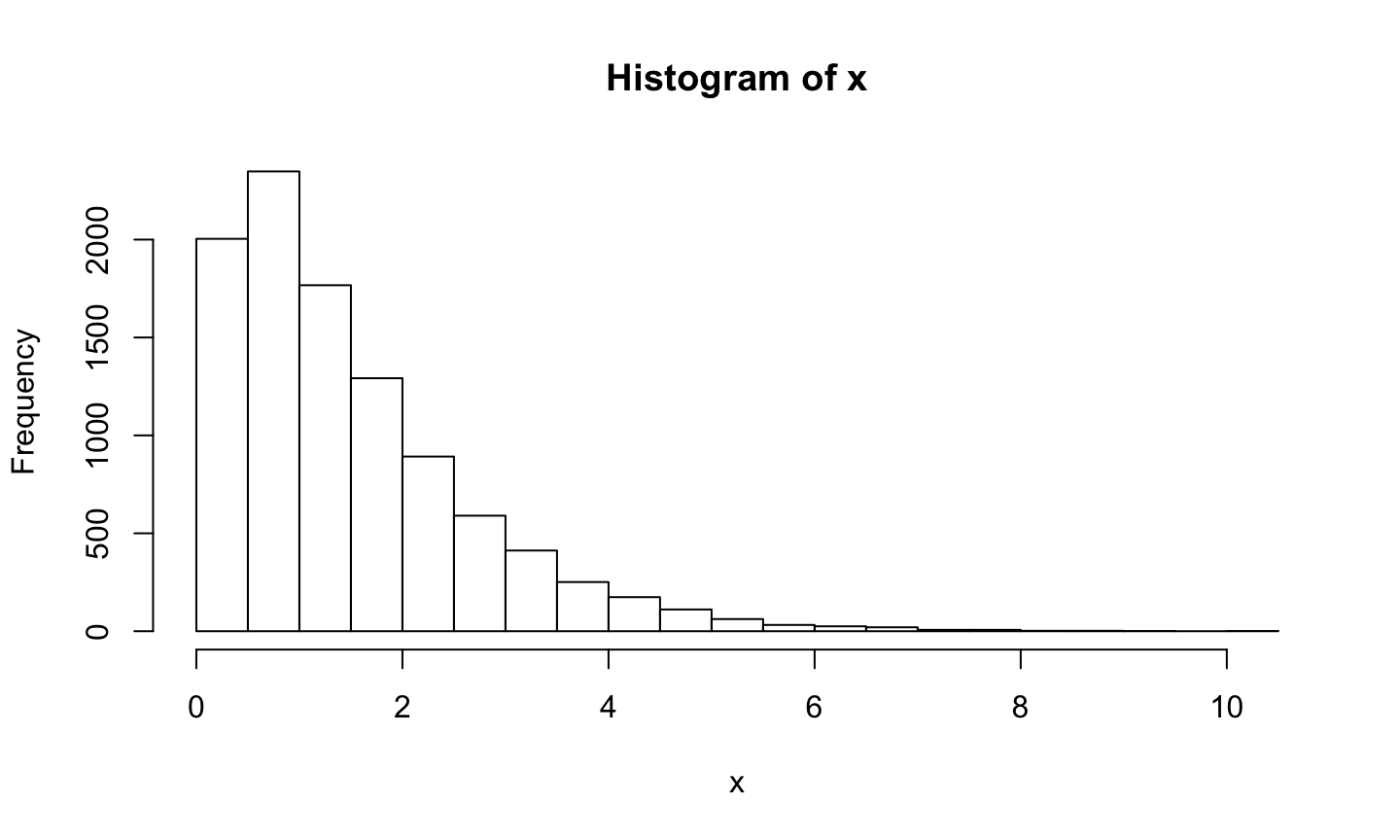mean(x)
##  1.482398

## Example: Gamma

true.x <- seq(0,10, .1)
true.y <- dgamma(true.x, 3/2, 1)
hist(x, freq=F, breaks=30, xlab="x", ylab="f(x)", main="Histogram and Theoretical")
points(true.x, true.y, type="l", col="red", lw=2)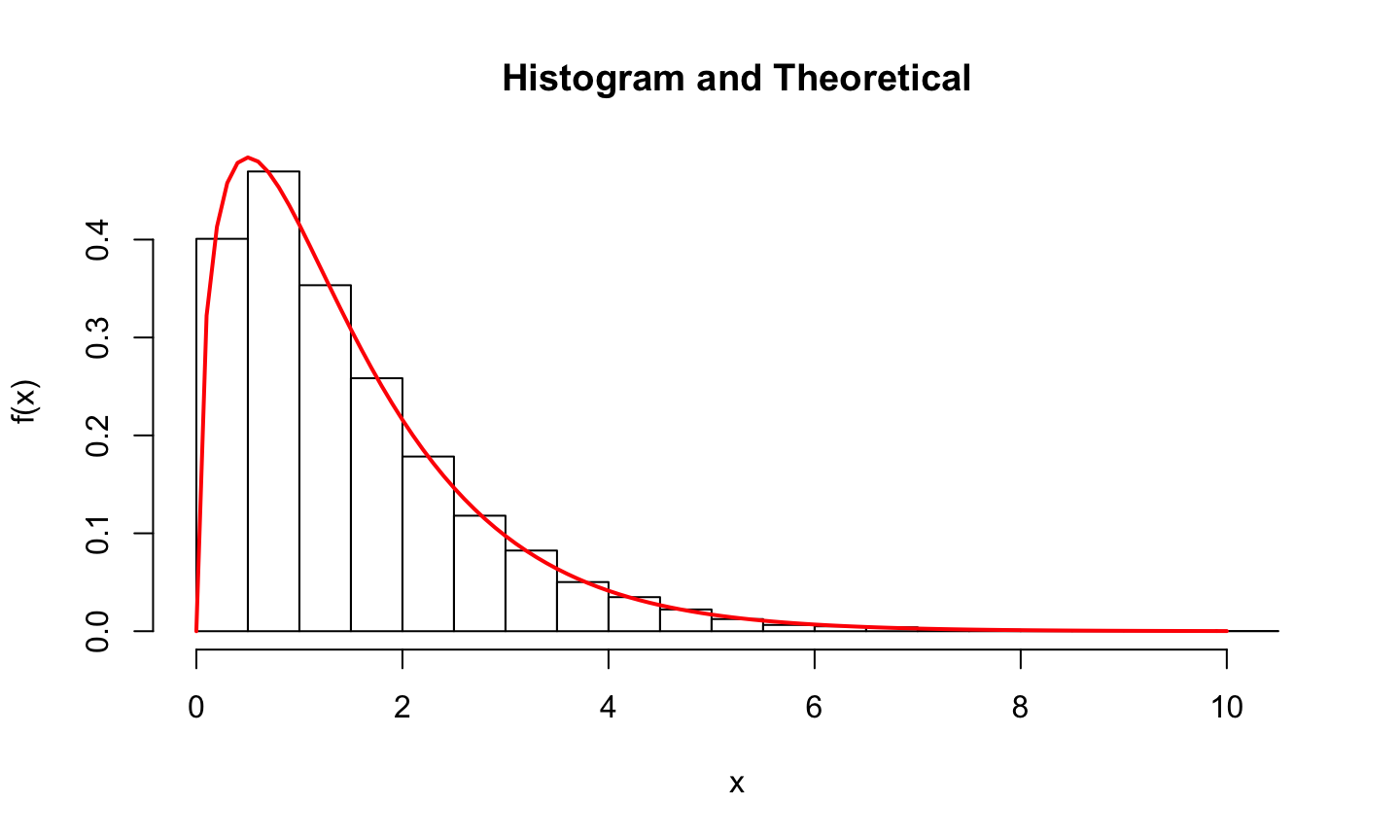## Example: Beta

Suppose the pdf $$f$$ is zero outside an interval $$[c,d]$$, and $$\leq M$$ on the interval.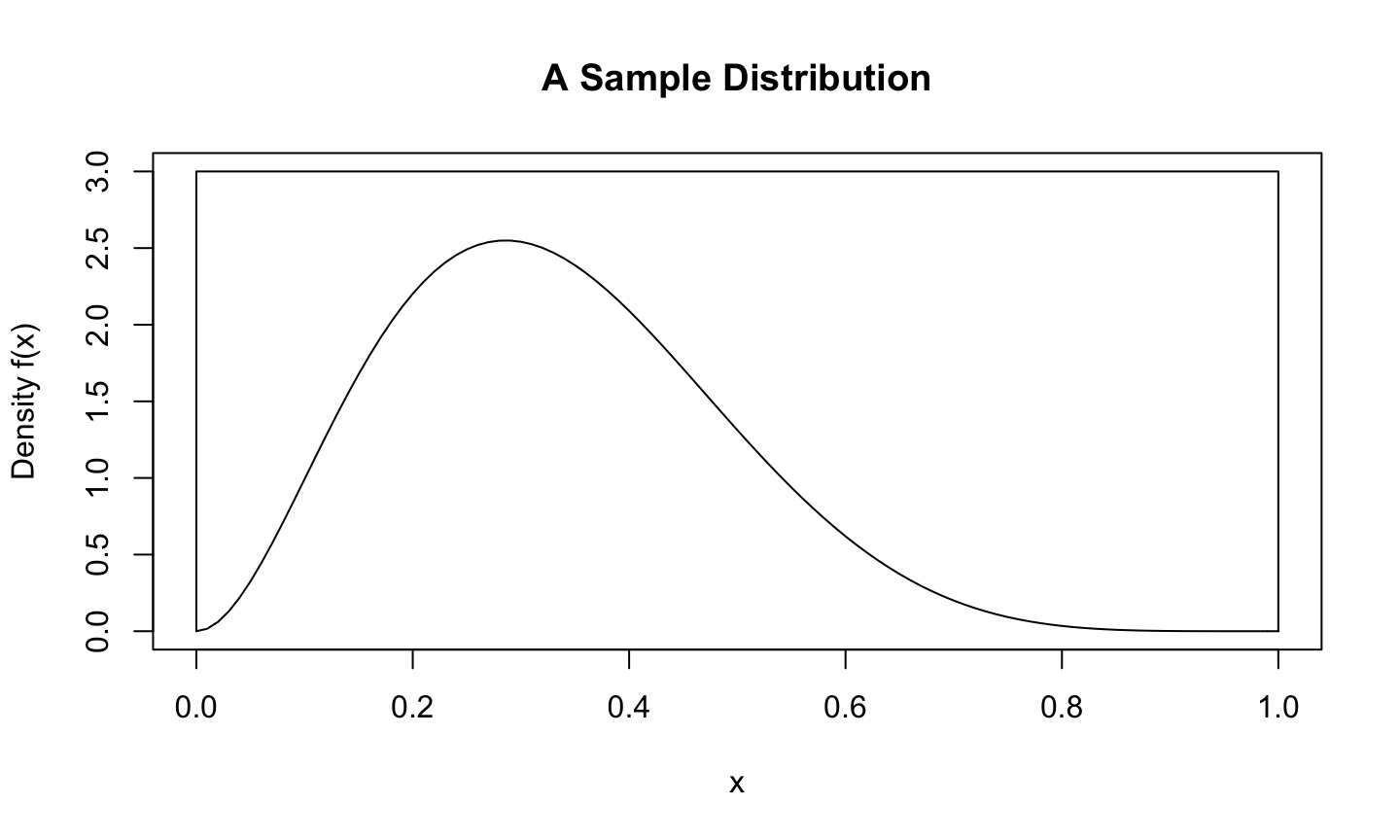## Example: Beta

We know how to draw from uniform distributions in any dimension. Do it in two:

x1 <- runif(300, 0, 1); y1 <- runif(300, 0, 2.6);
selected <- y1 < dbeta(x1, 3, 6)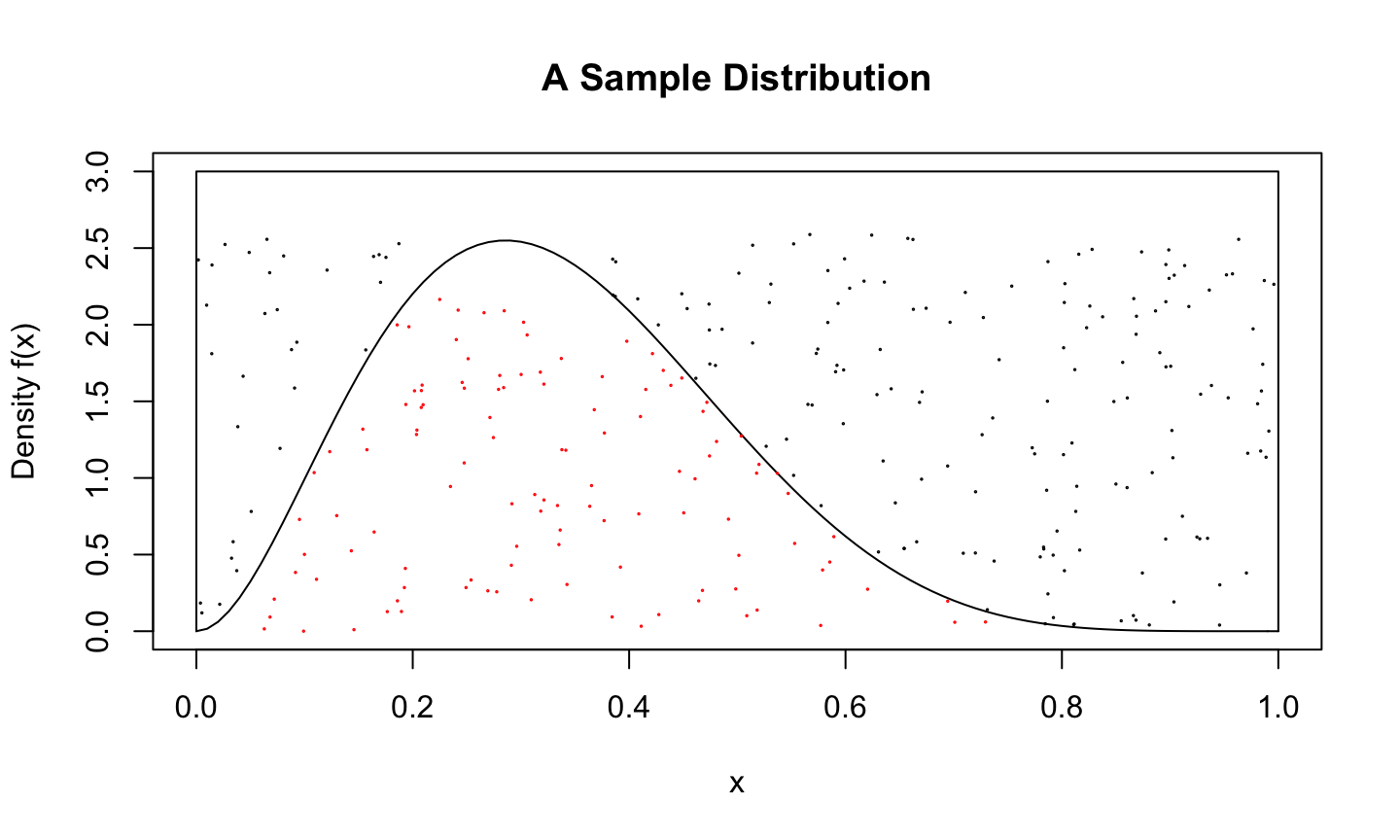## Example: Beta

mean(selected)
##  0.3833333
accepted.points <- x1[selected]
mean(accepted.points < 0.5)
##  0.8521739
pbeta(0.5, 3, 6)
##  0.8554688

## Example: Beta

For this to work efficiently, we have to cover the target distribution with one that sits close to it.

x2 <- runif(100000, 0, 1); y2 <- runif(100000, 0, 10);
selected <- y2 < dbeta(x2, 3, 6)
mean(selected)
##  0.09907